﻿ 惯性/星光组合系统高精度水平基准构造技术研究

# 惯性/星光组合系统高精度水平基准构造技术研究Study on High-Precision Horizontal Reference Construction Technology of INS/CNS

Abstract: Inertial navigation, as a reliable means that can provide navigation information in a certain period of time, can guarantee accuracy, but due to the influence of inertial device drift, its navigation accuracy is difficult to meet the needs of ship-borne weapons when long-haul. This paper discusses the inertial/starlight navigation technology, using the boundary characteristics of starlight navigation error to overcome the shortcomings of inertial navigation error with time, while the inertial guidance will continue to provide high-precision horizontal reference to the starlight navigation system, using complementary means based on the position and attitude information of the starlight system, to solve the problem of starlight navigation accuracy subject to inertial horizontal reference, to construct high-precision horizontal reference, to ensure the boundary of starlight error, so as to ensure the accuracy of starlight measurement information.

1. 引言

1) 视场较小，如JPL的ASTROS星敏感器视场为2.2˚ × 3.3˚；2) 姿态精度较低，如JPL的ASTROS星敏感器纵摇和航行精度为4″，横摇精度为50″；3) 更新率较低，小于≤10 Hz；4) 星敏感器的体积、质量和功耗较大；5) 不具备独立自主获取载体惯性系姿态的能力。

1) 视场较大，德国Jena-Optronik的ASTRO15星敏感器视场为13.3˚ × 13.3˚；2) 姿态精度较高，如德国Jena-Optronik的ASTRO15星敏感器纵摇和航行精度为1″，横摇精度为10″；3) 更新率较低，小于≤10 Hz；4) 体积小，重量轻，功耗低；5) 具有独立自主获取载体惯性系姿态的能力。

1) 更大的视场，德国Jena-Optronik的ASTRO APS星敏感器视场为20˚ × 20˚；2) 较高的姿态精度，如德国Jena-Optronik的ASTRO APS星敏感器纵摇和航行精度为1″，横摇精度为8″；3) 更新率较高，德国Jena-Optronik的ASTRO APS星敏感器的更新率高达104 Hz；4) CMOS APS探测器集成度较高，因此具有极低的功耗、更小的体积和重量；5) 可实现红外波段探测功能。

2. 惯性/星光坐标系及其关系

1) 地心惯性坐标系(i系)

2) 地球坐标系(e系)

3) 地理坐标系(t系)

4) 载体坐标系(b系)

5) 平台坐标系(p系)

6) 导航坐标系(n系)

7) 星敏感器坐标系(s系)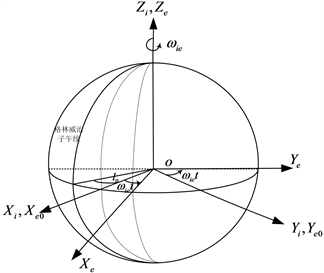Figure 1. Diagram of relationship between earth coordinate and geocentric inertial coordinateFigure 2. Star sensor measurement coordinate system (s coordinate)

3. 惯性/星光导航方案

3.1. 船舶平台星光特点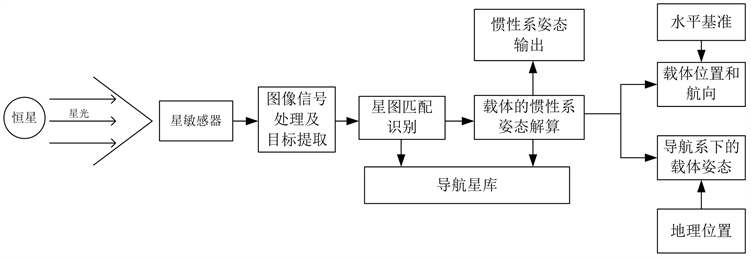Figure 3. Schematic diagram of star navigation mechanical arrangement

1) 采用多孔径星体敏感器技术，Daystar和Daytime Stellarimager均通过三个独立的星体捕获、成像与检测单元增强观星效果；

2) 采用红外探测器，满足白天海上观星导航的要求；

3) 具有较强的白天测星能力，最高白天测星能力达到+7.1等；

4) 定位精度高，Daytime Stellarimager定位精度优于200 m；

5) 采用固态形式，捷联系统的组合方式，提高系统的可靠性。

3.2. 船舶平台惯导特点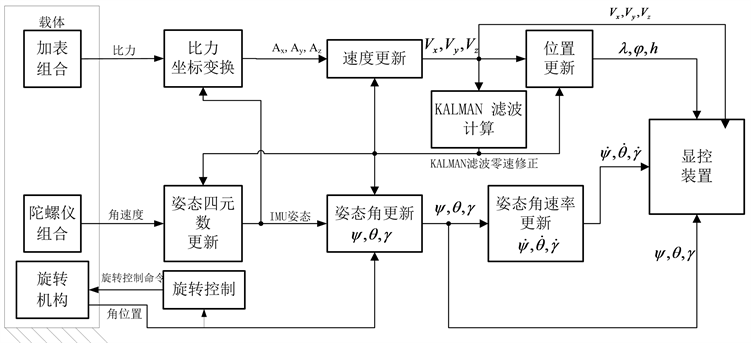Figure 4. Mechanical schematic diagram of single axis rotary strapdown inertial navigation system

3.3. 惯性/星光组合特点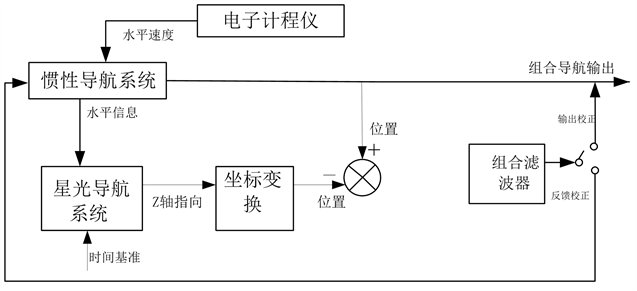Figure 5. INS/CNS combination mode

4. 高精度水平姿态基准构造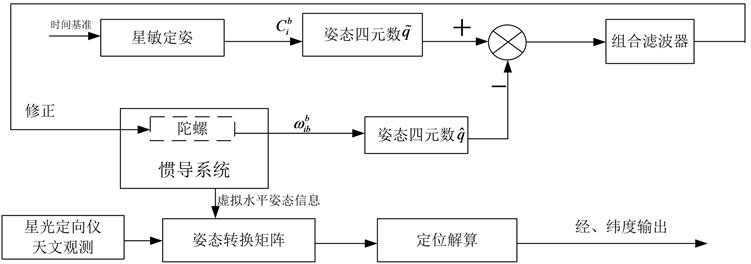Figure 6. Construction scheme of high precision horizontal attitude reference

${\stackrel{˙}{q}}_{i/b}=0.5{q}_{i/b}\otimes {\omega }_{i/b}^{b}$ (1)

$Q\left({\stackrel{˙}{q}}_{i/b}\right)=0.5M\left({q}_{i/b}\right)\otimes Q\left({\omega }_{i/b}^{b}\right)=0.5{M}^{\ast }\left({\omega }_{i/b}^{b}\right)Q\left({q}_{i/b}\right)$ (2)

$Q\left({\stackrel{˙}{q}}_{i/b}\right)=0.5{M}^{\ast }\left({\omega }_{i/b}^{b}\right)Q\left({q}_{i/b}\right)$ 两边同时微分，得四元数为状态变量的误差方程：

$Q\left(\delta {\stackrel{˙}{q}}_{i/b}\right)=0.5{M}^{\ast }\left({\omega }_{i/b}^{b}\right)Q\left(\delta {q}_{i/b}\right)+0.5{M}^{\ast }\left({q}_{i/b}\right)Q\left(\delta {\omega }_{i/b}^{b}\right)$ (3)

$\delta {q}_{i/b}$ ——姿态四元数误差：

$\delta {q}_{i/b}={\stackrel{˜}{q}}_{i/b}-{\stackrel{^}{q}}_{i/b}$ (4)

$\delta {\omega }_{i/b}^{b}$ ——陀螺角速度误差：

$\begin{array}{l}\delta {\omega }_{i/b}^{b}={\epsilon }_{b}+{\epsilon }_{r}+{\epsilon }_{g}\\ \left\{\begin{array}{l}{\stackrel{˙}{\epsilon }}_{b}=0\\ {\stackrel{˙}{\epsilon }}_{r}=-\frac{1}{{T}_{g}}{\epsilon }_{r}+{w}_{r}\end{array}\end{array}$ (5)

${\epsilon }_{b}$ ——随机常数；

${\epsilon }_{r}$ ——一阶马尔可夫过程随机噪声；

${\omega }_{g}$ ——白噪声；

${T}_{g}$ ——陀螺漂移的一阶马尔柯夫过程的相关时间。

$\stackrel{˙}{X}=F\left(t\right)X\left(t\right)+G\left(t\right)W\left(t\right)$ (6)

$F\left(t\right)$ 为系统状态矩阵， $G\left(t\right)$ 为系统噪声矩阵，定义分别如下：

$\begin{array}{l}F\left(t\right)={\left(\begin{array}{ccc}0.5{M}^{\ast }{\left({\omega }_{ib}^{b}\right)}_{4×4}& 0.5{M}^{\prime }{\left({q}_{i/b}\right)}_{4×3}& 0.5{M}^{\prime }{\left({q}_{i/b}\right)}_{4×3}\\ & {0}_{3×10}& \\ {0}_{3×7}& & {F}_{M3×3}\end{array}\right)}_{10×10}\\ G\left(t\right)={\left(\begin{array}{ccc}0.5{M}^{\prime }{\left({q}_{i/b}\right)}_{4×3}& \cdots & {0}_{4×7}\\ ⋮& \ddots & ⋮\\ {0}_{6×7}& \cdots & {I}_{6×3}\end{array}\right)}_{10×10}\end{array}$

$Z={\stackrel{˜}{q}}_{i/b}-{\stackrel{^}{q}}_{i/b}=\delta {q}_{i/b}=HX+V$ (7)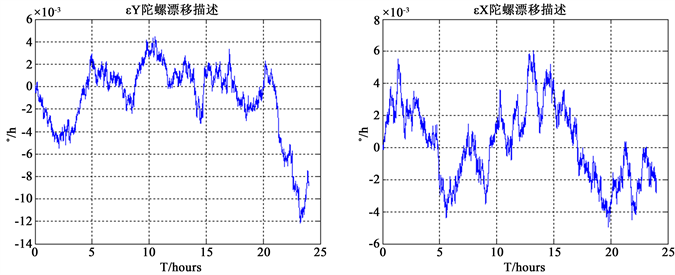Figure 7. First order Markov process description of horizontal gyroscope (X, Y)Figure 8. First order Markov process description of vertical gyroscope (Z)Figure 9. Comparison of roll error between INS and INS/CNS attitude combinations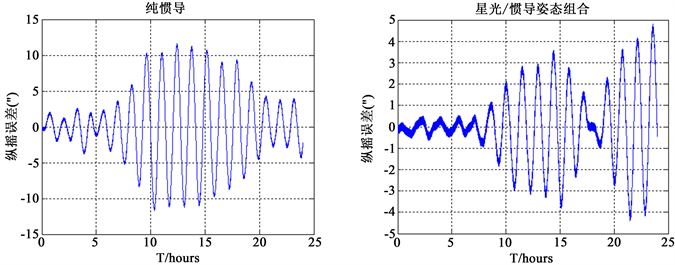Figure 10. Comparison of pitch error between INS and INS/CNS attitude combinations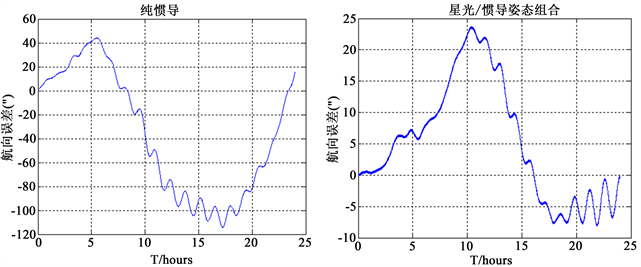Figure 11. Comparison of head error between INS and INS/CNS attitude combinations

5. 结论

 王振华. 天文导航技术与装备的发展现状及对策[J]. 光学与光电技术. 2005, 3(5): 7-13.

 王鹏, 张迎春. 基于SINS/星敏感器的组合导航模式[J]. 东南大学学报(自然科学版), 2005(2): 84-89.

 张金亮. 捷联惯性/星光组合导航关键技术研究[D]: [博士学位论文]. 西安: 西北工业大学, 2016: 2-5.

 谭汉清, 刘垒. 惯性/星光组合导航技术综述[J]. 控制与制导, 2008(5): 44-51.

 Aghaei, M. and Moghaddam, H.A. (2016) Grid Star Identification Improvement Using Optimization Approaches. IEEE Transition Aerospace Electronic System, 52, 2080-2090.
https://doi.org/10.1109/TAES.2016.150053

 Zhang, C., Niu, Y., Zhang, H. and Lu, J. (2018) Optimized Star Sensors Laboratory Calibration Method Using a Regularization Neural Network. Applied Optics, 57, 1067-1074.
https://doi.org/10.1364/AO.57.001067

 茅永兴, 张同双, 朱伟康, 等. 基于星惯组合的分布式船姿船位测量体制研究[J]. 飞行器测控学报, 2013, 32(1): 23-26.

 Mortari, D., Pollock, T.C. and Junkins, J.L. (1998) Towards the Most Accurate Attitude Determination System Using Star Trackers. Advances in the Astronautical Sciences, 99, 839-850.

 Majewski, L., Blarre, L., Perrimon, N., Kocher, Y., Martinez, P.E. and Dussy, S. (2008) HYDRA Multiple Head Star Tracker with Enhanced Performance. 7th International ESA Conference on Guidance, Navigation & Control Systems, Tralee, 2-5 June 2008.

 Zhou, L., Dong, Y., Zhao, W., Zhao, X., Qu, Y. and Hou, Z. (2016) Novel SCNS/RSINS Tight-Integrated Alignment Based on Adaptive Interacting Multiple Model Filters on Shipboard. Journal of Chinese Inertial Technology, 24, 464-472.

 周凌峰, 赵小明, 赵帅, 等. 基于递推最小二乘估计的CNS/INS组合导航系统初始对准[J]. 中国惯性技术学报, 2015, 23(3): 281-286.

 朱长征. 基于星敏感器的星模式识别算法及空间飞行器姿态确定技术研究[D]: [博士学位论文]. 长沙: 国防科学技术大学, 2004: 1-10.

Top